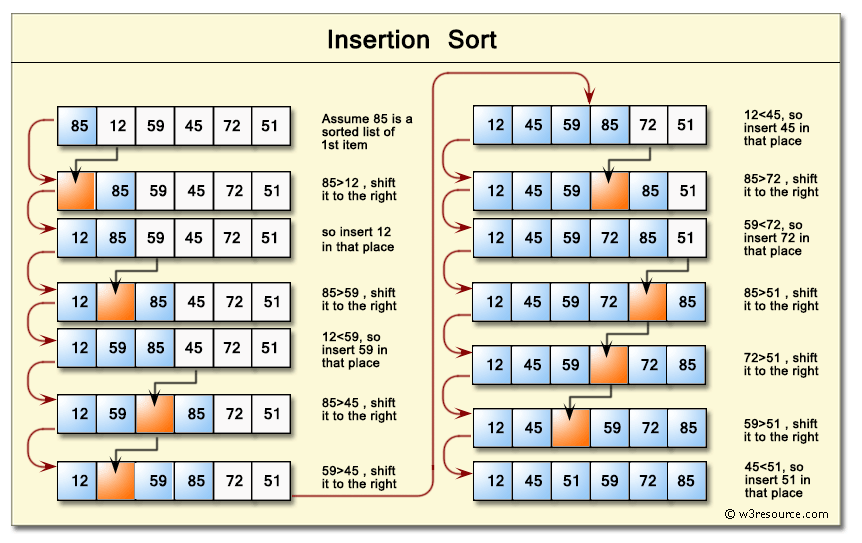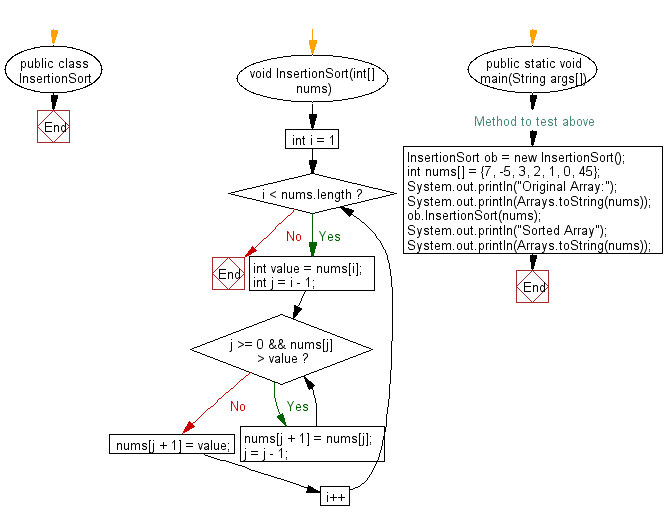﻿ Java exercises: Insertion sort Algorithm - w3resource# Java Exercises: Insertion sort Algorithm

## Java Sorting Algorithm: Exercise-7 with Solution

Write a Java program to sort an array of given integers using Insertion sort Algorithm.

Note:
Insertion sort is a simple sorting algorithm that builds the final sorted array (or list) one item at a time. It is much less efficient on large lists than other algorithms such as quicksort, heapsort, or merge sort.

Pictorial presentation - Insertion search algorithm :Sample Solution:

Java Code:

``````import java.util.Arrays;

public class InsertionSort {
void InsertionSort(int[] nums){
for(int i = 1; i < nums.length; i++){
int value = nums[i];
int j = i - 1;
while(j >= 0 && nums[j] > value){
nums[j + 1] = nums[j];
j = j - 1;
}
nums[j + 1] = value;
}
}

// Method to test above
public static void main(String args[])
{
InsertionSort ob = new InsertionSort();
int nums[] = {7, -5, 3, 2, 1, 0, 45};
System.out.println("Original Array:");
System.out.println(Arrays.toString(nums));
ob.InsertionSort(nums);
System.out.println("Sorted Array");
System.out.println(Arrays.toString(nums));
}
}
``````

Sample Output:

```[7, -5, 3, 2, 1, 0, 45]
Sorted Array
[-5, 0, 1, 2, 3, 7, 45]
```

Flowchart:Java Code Editor:

What is the difficulty level of this exercise?

﻿

## Java: Tips of the Day

Array vs ArrayLists:

The main difference between these two is that an Array is of fixed size so once you have created an Array you cannot change it but the ArrayList is not of fixed size. You can create instances of ArrayLists without specifying its size. So if you create such instances of an ArrayList without specifying its size Java will create an instance of an ArrayList of default size.

Once an ArrayList is full it re-sizes itself. In fact, an ArrayList is internally supported by an array. So when an ArrayList is resized it will slow down its performance a bit as the contents of the old Array must be copied to a new Array.

At the same time, it's compulsory to specify the size of an Array directly or indirectly while creating it. And also Arrays can store both primitives and objects while ArrayLists only can store objects.

Ref: https://bit.ly/3o8L2KH